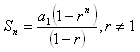Geometric

Adding the terms of the sequence is required to solve many applications of sequences. The sum of the terms of a sequence is called a series. A geometric series is the sum of the terms of a geometric sequence. For example, the sum of the first five terms of a geometric sequence is represented by:

S5 = a1 + a2 + a3 + a4 + a5

In general, the sum of the first n terms of a geometric sequence is:

Sn = a1 + a2 + a3 + a4 + ... + an

For a geometric sequence with first term a1, a common ratio of r, and n terms the series is given by the formula: